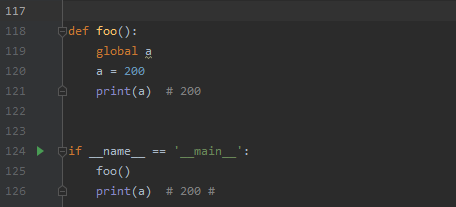# problem c: 判断素数的函数_python100天 — Day 6 函数和模块的使用﻿> 本文以GitHub上100天python从新手到大师为素材，规划为约100天的练习，每次的学习和总结均发在这里。有错误的地方还请朋友们指正。

## 函数的作用

a = float(input("输入第一条边的长度： "))
b = float(input("输入第二条边的长度： "))
c = float(input("输入第三条边的长度： "))

if a + b > c and a + c > b and b + c > a:
perimeter = a + b + c
p = perimeter / 2
area = (p * (p - a) * (p - b) * (p - c))**0.5
print("perimeter is %.2f narea is %.2f" %(perimeter,area))
else:
print("该边长组不成立三角形")

def tri_area(a,b,c):
if a + b > c and a + c > b and b + c > a:
perimeter = a + b + c
p = perimeter / 2
area = (p * (p - a) * (p - b) * (p - c)) ** 0.5
return area
else:
print('无法计算面积')

print(tri_area(2,4,5))
print(tri_area(3,4,5))
print(tri_area(2,4,7))

## 函数的参数

from random import randint

def Throw_the_dice(n=2):
total = 0
for i in range(n):
total += randint(1,6)
return total

def add(*args):
total = 0
for i in args:
total += i
return  total

*args 可以承载多个数据传入函数中，例如：

print(add(1,2,3))

## 用模块管理函数

def foo():
print("hello python")

def foo():
print("hello world")

print(foo())

#module2.py#
def foo():
print("hello python")

#module3.py#
def foo():
print("hello world")

from module2 import foo
foo()

from module3 import foo
foo()

#或者#

import module2 as md2
import module3 as md3
m2.foo()
m3.foo()

from module2 import foo
from module3 import foo
foo()
foo()

#module3.py#
print("干扰文字")

def foo():
print(foo())

def foo():
print("Hello World")

if __name__ == "__mian__":
print("干扰文字")

## 练习部分

### 实现计算最大公约数和最小公倍数

def gcd_lcd(x,y):
if x > y:
x ,y = y, x #保证x比y小#
gcd_num = 1
for i in range(x,0,-1):
if x%i == 0 and y%i == 0:
gcd_num = i
break
lcd = x * y / gcd_num
return gcd_num, lcd

def gcd(x, y):
"""求最大公约数"""
(x, y) = (y, x) if x > y else (x, y)
for factor in range(x, 0, -1):
if x % factor == 0 and y % factor == 0:
return factor

def lcm(x, y):
"""求最小公倍数"""
return x * y // gcd(x, y)

### 判断一个数是不是回文数

Tips：回文数即从前往后和往后往前看时一样的

def palindromic(num):
sum_total = 0
here = num
while True:
sum_total = sum_total*10 + (num % 10)
if num // 10 == 0:
break
else:
num = num//10

return  sum_total == here

def is_palindrome(num):
"""判断一个数是不是回文数"""
temp = num
total = 0
while temp > 0:
total = total * 10 + temp % 10
temp //= 10
return total == num

### 判断一个数是不是素数

def is_prime(num):
kaigen = int(num**0.5)+1
test_num = 2
not_prime = True
while not_prime:
if num % test_num == 0 and num > 1 :
return False
not_prime = False
elif test_num < kaigen:
test_num += 1
else:
return True
break

def is_prime(num):
"""判断一个数是不是素数"""
for factor in range(2, num):
if num % factor == 0:
return False
return True if num != 1 else False

### 判断输入的正整数是不是回文素数

def alltrue(num):
if is_prime(num) and palindromic(num):
return True
else:
return False

if __name__ == '__main__':
num = int(input('请输入正整数: '))
if is_palindrome(num) and is_prime(num):
print('%d是回文素数' % num)

## 变量作用域

def foo():
a = 100
print(a)  # 200

if __name__ == "__main__":
a = 200
foo()

def foo():
b = 'hello'

# Python中可以在函数内部再定义函数
def bar():
c = True
print(a)
print(b)
print(c)

bar()
# print(c)  # NameError: name 'c' is not defined

if __name__ == '__main__':
a = 100
# print(b)  # NameError: name 'b' is not defined
foo()

def foo():
global a
a = 200
print(a)  # 200

if __name__ == '__main__':
foo()
print(a)  # 200

11-244205-21602
10-1241
11-04144
03-052923
11-06215
08-053万+
02-2444
09-281万+
03-01141
05-1380
03-018
09-08139
06-05149
01-0934
09-01424
09-08662
12-3138
©️2020 CSDN 皮肤主题: 数字20 设计师:CSDN官方博客